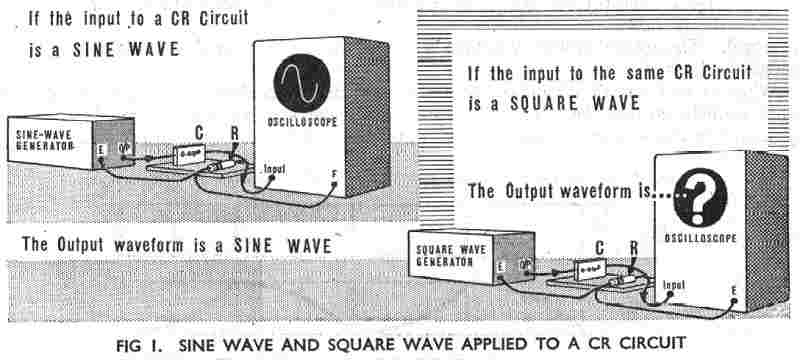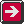Main Radar Home AP3302 Pt3 Contents AP3302 Pt3 Section 2Contents AP 3302 Pt. 3 Section 2 CHAPTER 2 Square Waves applied to CR circuits Introduction In Chapter 1 we showed that square and rectangular waves may be used to switch radar circuits on and off at precise instants of time. However, square waves are used in radar for many other purposes also. Many radar circuits actually depend for their operation upon the effects of applying a square wave to a capacitor-resistor (CR) circuit. We already know that if a sine wave input is applied to a CR circuit the output waveform is also a sine wave (Fig I). However if we apply a square wave input to a CR circuit the output is not necessarily a square wave. In this chapter we shall examine the effects of applying square waves to CR circuits.CR Time Constant We already know from Part I of these notes that when a resistor R is connected in series with a capacitor C across a source of voltage: a. The charge on the capacitor cannot change instantaneously so that initially the full source voltage is developed across R. b. The capacitor then commences to charge exponentially at a rate dependent upon the circuit time constant, CR seconds. As the voltage Vc across rises so the voltage Vr across R falls. c. After a time equal to CR seconds the capacitor is two-thirds (63 per cent) charged and is assumed to be fully charged after 5 CR seconds. d. At all times the sum of the voltages across C and R equals the applied voltage (Kirchhoff's second law), i.e. Vc+Vr = V. Similarly when C is allowed to discharge through R, Vc falls exponentially from the charged voltage to zero in a time of 5 CR seconds. Thus when we apply a square wave of voltage to a CR circuit, Vc will rise and fall exponentially and Vr will vary correspondingly to maintain the relationship Vc + Vr = V. Let us now consider this action in more detail. Effect of Applying a Square Wave to a CR Circuit In Fig 2 we have a CR circuit and a simple "square wave generator", i.e. the battery and the switch. Let us start from a point when the switch is in the discharge position and C is fullyPrevious page To top of this page Next Page

Constructed by Dick Barrett
Email: